Courses

# Universal Velocity Distribution Law & Friction Factor in Duct Flows - 1 Civil Engineering (CE) Notes | EduRev

## Civil Engineering (CE) : Universal Velocity Distribution Law & Friction Factor in Duct Flows - 1 Civil Engineering (CE) Notes | EduRev

The document Universal Velocity Distribution Law & Friction Factor in Duct Flows - 1 Civil Engineering (CE) Notes | EduRev is a part of the Civil Engineering (CE) Course Fluid Mechanics.
All you need of Civil Engineering (CE) at this link: Civil Engineering (CE)

Universal Velocity Distribution Law And Friction Factor In Duct Flows For Very Large Reynolds  Number

• For flows in a rectangular channel at very large Reynolds numbers the laminar sublayer can practically be ignored. The channel may be assumed to have a width 2h and the x axis will be placed along the bottom wall of the channel.

• Consider a turbulent stream along a smooth flat wall in such a duct and denote the distance from the bottom wall by y, while u(y) will signify the velocity. In the neighbourhood of the wall, we shall apply

l = x y

• According to Prandtl's assumption, the turbulent shearing stress will be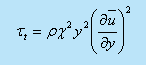( 34.1)

At this point, Prandtl introduced an additional assumption which like a plane Couette flow takes a constant shearing stress throughout, i.e

τt = τw                                                                                                        ( 34.2)

where tw   denotes the shearing stress at the wall.

• Invoking once more the friction velocity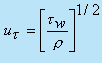, we obtain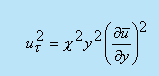(34.3)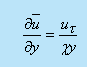(34.4)

On integrating we find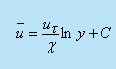(34.5)

Despite the fact that Eq. (34.5) is derived on the basis of the friction velocity in the neighbourhood of the wall because of the assumption that Tw = Tt constant, we shall use it for the entire region. At y = h (at the horizontal mid plane of the channel), we have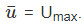The constant of integration is eliminated by considering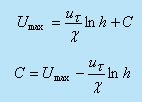Substituting C in Eq. (34.5), we get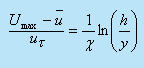(34.6)

Equation (34.6) is known as universal velocity defect law of Prandtl and its distribution has been shown in Fig. 34.1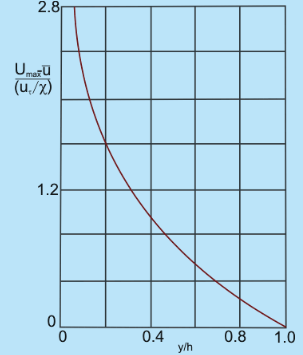Fig 34.1 Distibution of universal velocity defect law of Prandtl in a turbulent channel flow

Here, we have seen that the friction velocity ur is a reference parameter for velocity.Equation (34.5) can be rewritten as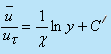where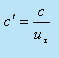.Contd. from previous slide

The no-slip condition at the wall cannot be satisfied with a finite constant of integration. This is expected that the appropriate condition for the present problem should be that y0 at a very small distance y= y0 from the wall. Hence, Eq. (34.5) becomes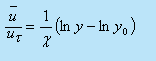• The distance y0  is of the order of magnitude of the thickness of the viscous layer. Now we can write Eq. (34.7) as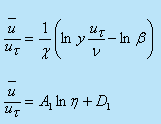where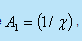the unknown β is included in D1 .

Equation (34.8) is generally known as the universal velocity profile because of the fact that it is applicable from moderate to a very large Reynolds number.

However, the constants A1 and D1 have to be found out from experiments. The aforesaid profile is not only valid for channel (rectangular) flows, it retains the same                 functional relationship for circular pipes as well . It may be mentioned that even without the assumption of having a constant shear stress throughout, the universal                     velocity profile can be derived

• Experiments, performed by J. Nikuradse, showed that Eq. (34.8) is in good agreement with experimental results. Based on Nikuradse's and Reichardt's experimental data, the empirical constants of Eq. (34.8) can be determined for a smooth pipe as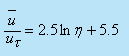(34.9)

This velocity distribution has been shown through curve (b) in Fig. 34.2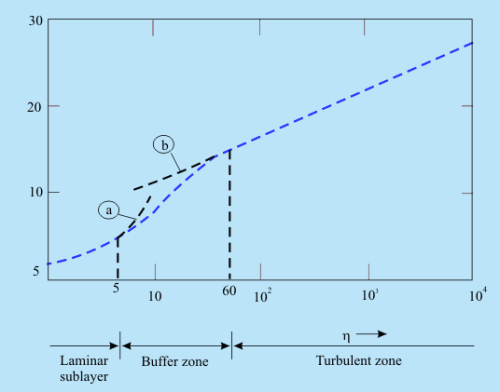Fig 34.2   The universal velocity distribution law for smooth pipes

• However, the corresponding friction factor concerning Eq. (34.9) is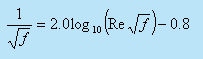The universal velocity profile does not match very close to the wall where the viscous shear predominates the flow

• Von Karman suggested a modification for the laminar sublayer and the buffer zone which are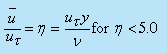(34.11)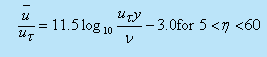(34.12)

Equation (34.11) has been shown through curve(a) in Fig. 34.2.

• It may be worthwhile to mention here that a surface is said to be hydraulically smooth so long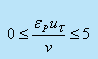(34.13)

where ∈p is the average height of the protrusions inside the pipe.

Physically, the above expression means that for smooth pipes protrusions will not be extended outside the laminar sublayer. If protrusions exceed the thickness of laminar sublayer, it is conjectured (also justified though experimental verification) that some additional frictional resistance will contribute to pipe friction due to the form drag experienced by the protrusions in the boundary layer.

• In rough pipes experiments indicate that the velocity profile may be expressed as: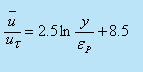(34.14)

At the centre-line, the maximum velocity is expressed as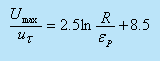(34.15)

Note that v no longer appears with R and p . This means that for completely rough zone of turbulent flow, the profile is independent of Reynolds number and a strong function of pipe roughness .

• However, for pipe roughness of varying degrees, the recommendation due to Colebrook and White works well. Their formula is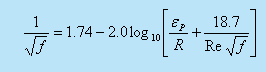(34.16)

where R  is the pipe radius

For →0 , this equation produces the result of the smooth pipes (Eq.(34.10)). For Re→∞ , it gives the expression for friction factor for a completely rough pipe at a very high Reynolds number which is given by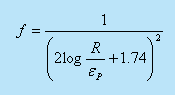(34.17)

Turbulent flow through pipes has been investigated by many researchers because of its enormous practical importance.

Fully Developed Turbulent Flow In A Pipe For Moderate Reynolds Numbers

• The entry length of a turbulent flow is much shorter than that of a laminar flow, J. Nikuradse determined that a fully developed profile for turbulent flow can be observed after an entry length of 25 to 40 diameters. We shall focus to fully developed turbulent flow in this section.
• Considering a fully developed turbulent pipe flow (Fig. 34.3) we can write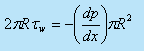(34.18)

or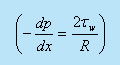(34.19)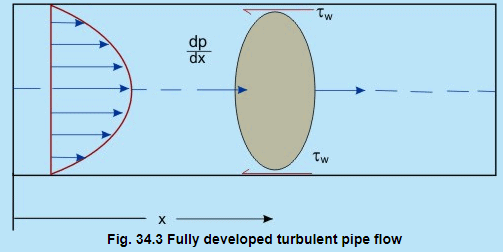It can be said that in a fully developed flow, the pressure gradient balances the wall shear stress only and has a constant value at any x. However, the friction factor ( Darcy friction factor ) is defined in a fully developed flow as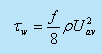(34.20)

Comparing Eq.(34.19) with Eq.(34.20), we can write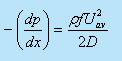(34.21)

H. Blasius conducted a critical survey of available experimental results and established the empirical correlation for the above equation as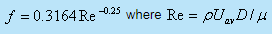(34.22)

• It is found that the Blasius's formula is valid in the range of Reynolds number of Re ≤105. At the time when Blasius compiled the experimental data, results for higher Reynolds numbers were not available. However, later on, J. Nikuradse carried out experiments with the laws of friction in a very wide range of Reynolds numbers, 4 x 103 ≤ Re ≤ 3.2 x 106. The velocity profile in this range follows: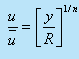(34.23)

where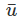is the time mean velocity at the pipe centre and y is the distance from the wall . The exponent varies slightly with Reynolds number. In the range of Re ~ 105, n is 7.

Offer running on EduRev: Apply code STAYHOME200 to get INR 200 off on our premium plan EduRev Infinity!

## Fluid Mechanics

43 videos|70 docs|72 tests

,

,

,

,

,

,

,

,

,

,

,

,

,

,

,

,

,

,

,

,

,

;